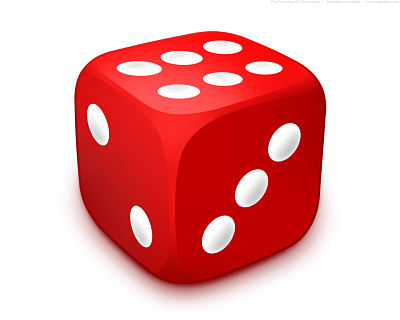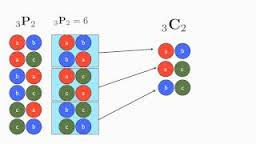With elite exams like the JEE and BITSAT just a few days away, students are busy doing their last minute preparation. Its high time that the budding engineers steer their focus on something beyond preparation. Now is the time to gear up for revision and to brush up your concepts. Prestigious exams like the JEE and BITSAT test in depth knowledge of the students along with analytical skills. It is thus necessary for the aspirants to have strong basics and a clear understanding of concepts.

Permutations and Combinations is one of the chapters in the syllabus of IIT JEE, which holds a place of importance and is also simple to understand. The topic on Permutations and Combinations is also extremely useful as it is a pre-requisite for the chapter on Probability. It also lays the foundation for Binomial theorem. The topic is generally considered to be tricky as students fail to understand the difference between the two concepts. We consider both the topics one by one:

Permutation: A permutation is a one Ã¢â‚¬â€œ to Ã¢â‚¬â€œ one correspondence of a set onto itself. It is basically an arrangement of a set of objects in a particular order. The total number of permutations possible on a set of n objects is given by n factorial written as n! The number of permutations of n objects, taken r at a time, is the total number of arrangements of n objects, in groups of r where the order of the arrangement is important.

Permutations may be with repetition or without repetition. The formula in both the cases differs slightly.

(i) Without repetition

(a) Arranging n objects, taking r at a time in every arrangement, is equivalent to filling r places from n things.
Number of ways of arranging = Number of ways of filling r places
= n(n – 1)(n – 2) … (n – r + 1)
= (n(n – 1)(n – 2) … (n – r + 1)((n-r)!))/((n-r)!)
= n!/((n-r)!)Ã‚Â  = nPr.
(b) Number of arrangements of n different objects taken all at a time = npn = n!

(ii) With repetition

(a) Number of permutations (arrangements) of n different objects, taken r at a time, when each object may occur once, twice, thrice …… up to r times in any arrangement = Number of ways of filling r places, each out of n objects.
Hence, Number of ways to arrange = Number of ways to fill r places = (n)r.
(iii) Number of arrangements that can be formed using n objects out of which p are identical (and of one kind), q are identical (and of one kind) and rest are different = n!/p!q!r!.

Combinations:

Just like permutations, combination is also a way of selecting objects from a larger group, but without considering the order of objects. A combination is thus a selection of r items from a total of n items where order hasnÃ¢â‚¬â„¢t got any importance. The formula used for this computation is nCr = n!/ r!(n-r)!

(i) Selection of objects without repetition:
The number of selections (combinations or groups) that can be formed from n different objects taken r (0Ã‚Â <Ã‚Â rÃ‚Â <Ã‚Â n) at a time isÃ‚Â nCrÃ‚Â = n!/(r!(n-r))!.

(ii) Selection of objects with repetition:
If we need to compute the combination of n different items taken r at a time wherein the possibility of occurrence of any item may be once, twice or thriceÃ¢â‚¬Â¦.. up to r times, then it is calculated with the help of the formula n+r-1Cr.

Permutations vs. Combinations:
Permutations and combinations are quite inter-related but there is a slight difference between the two. The chief difference between the two concepts is that of order. When the order of items is important then permutations are used while if the order of items is not important then combination is used. A permutation in fact, may also be termed as an ordered combination.Click here for in-depth study of permutations and combinations.
Revision notes or last minute tips on permutations and combinations are just a click away.

Permutation and combination is also an important chapter of BITSAT as well as IIT JEE as questions are often asked from this chapter in the exam. Though the topic is quite vast but the examiners generally frame some standard questions. Questions are often asked on arrangement of letters of words. While attempting questions on these topics, first try to identify whether the order of things is important. Once you are able to judge whether the question is of permutation or combination, half the problem is solved. Then try to figure out whether it is the case of repetition or without repetition. And then apply the required formula.